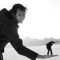# Pfarrmedientag 2019• Keine Tags gefunden...

PFARRMEDIENTAG

2019

D I Ö Z E S E S T . P Ö L T E N

2 8 . S E P T E M B E R 2 0 1 9 | 1 0 - 1 6 U H R

K O N S E R V A T O R I U M , K L O S T E R G A S S E 1 0 , 3 1 0 0 S T . P Ö L T E N

PROGRAMM

A N K O M M E N & A N M E L D U N G

0 9 : 0 0 A M - 0 9 : 4 5 A M

0 9 : 4 5 A M

G E M E I N S A M E S G E B E T

1 0 : 0 0 A M

B E G R Ü S S U N G

K E Y N O T E S P E A K E R

1 0 : 1 5 A M - 1 0 : 4 5 A M

W O R K S H O P S T E I L A

1 0 : 4 5 A M - 1 2 : 1 5 P M

1 2 : 1 5 P M - 1 3 : 3 0 P M

M I T T A G E S S E N

W O R K S H O P S T E I L B

1 3 : 3 0 A M - 1 5 : 0 0 P M

Z E I T Z U M N E T W O R K I N G

1 5 : 0 0 P M - 1 5 : 4 5 P M

A B S C H L U S S & E I N L A D U N G

1 5 : 4 5 P M - 1 6 : 0 0 P M

P F A R R M E D I E N W E T T B E W E R B

Ähnliche Magazine